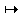# A Sticky Problem

The game presented by a Java applet below was published in 2002 the American Mathematical Monthly as problem #569 (Sung Soo Kim). A solution by Li Zhou appeared in v. 111, n. 4 (April, 2004), pp. 363-364.

A game starts with one stick of length 1 and four sticks of length 4. The two players move alternately. A move consists of breaking a stick of length at least two into two sticks of shorter lengths or removing n sticks of length n for some n{1, 2, 3, 4}. The player who makes the last move wins. Which player can force a win, and how?

The applet allows one to start with different initial configurations, but also with the standard one. At the outset, you can force the computer to make the first move by pressing the "Make move" button. Sticks are represented by a row of small squares that might remind of a chocolate brick. To break a piece click on a square to the right of the desired break line.

### This applet requires Sun's Java VM 2 which your browser may perceive as a popup. Which it is not. If you want to see the applet work, visit Sun's website at https://www.java.com/en/download/index.jsp, download and install Java VM and enjoy the applet.

 What if applet does not run?

StrategyEvery position in the game is completely determined by a quadruple (k, l, m, n), in which position #i indicates the number of sticks of length i.

Introduce

 r = (k + m) mod 2 and s = (l + n) mod 3.

We prove that the set P = {(k, l, m, n): r = s} is the set of winning positions. (Note that (0, 0, 0, 0)P.)

Let N be the set of positions not in P. It suffices to show that any move from a P-position leads to an N-position, and that for every N-position there is a move that leads to an P-position. (Note that (1, 0, 0, 4)P.)

A move that removes i sticks of length i reduces coordinate i by i; such a move changes exactly one of r or s. There are four possible stick-breaking moves:

 (1) (k, l, m, n)(k+2, l-1, m, n), (2) (k, l, m, n)(k+1, l+1, m-1, n), (3) (k, l, m, n)(k, l+2, m, n-1), (4) (k, l, m, n)(k+1, l, m+1, n-1).

Each of these also changes exactly one of r or s. Hence every move from a P-position leads to an N-position. From an N-position, the moves that lead to a P-position are listed below. Keep in mind that r is either 0 or 1, while s is either 0, or, 1, or 2.

N-Position Winning move s - r = -1 k(k - 1) if k > 0 (2) if m > 0 s - r = 1 (1) if l > 0 (3) if n > 0 s - r = 2 l(l - 2) if l > 1 (4) if n > 0

It remains to be shown that the specified moves are available. If k = m = 0, then r = 0, and s - r = -1 is impossible. If l = n = 0, then s = 0, and s - r = 1 is impossible. If n = 0 and l < 2, then s < 2, and s - r = 2 is impossible.• What Is a Combinatorial Game?
• A Game of Candy Squares
• Another Sticky Problem
• Date Game
• Dawson's Chess: an Interactive Gizmo
• Dawson's Kayles: an Interactive Gizmo
• Grundy's Game
• Hex 7
• Kayles
• Nimble: an Interactive Gizmo
• Northcott's game (An Interactive Gizmo)
• Odd Scoring
• One Pile: an Interactive Gizmo
• Plainim (An Interactive Gizmo)
• Plainim Misere (An Interactive Gizmo)
• Scoring: the simplest of the impartial games
• Scoring Misere: an Interactive Gizmo
• Scoring Misere: Two Heaps Perfect Strategy
• The Fraction Game
• The Silver Dollar Game
• Silver Dollar Game With No Silver Dollar
• Subtraction Game
• TacTix: an Interactive Gizmo
• Turning Turtles
• Take-Away Games
• Wythoff's Nim, Literal Implementation
• Wythoff's Nim (An Interactive Gizmo)
•